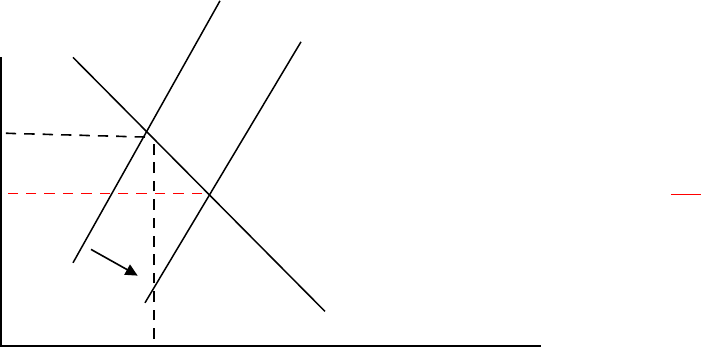Study Guides (390,000)
CA (150,000)
UTSC (10,000)
Midterm

# MGEB06H3 Study Guide - Midterm Guide: Nominal Interest Rate, Loanable Funds, Growth Accounting

Department
Economics for Management Studies
Course Code
MGEB06H3
Professor
Jack Parkinson
Study Guide
Midterm

This preview shows pages 1-3. to view the full 11 pages of the document.ECM B06 - WINTER 2011 – MIDTERM SOLUTIONS
Q1. (20 marks)
Solution
PART A:
LR Output
Y = 4(6,400)0.50(3,600)0.50 = 4(80)(60) = 19,200 (2 marks)
Real interest rate
Y = C + I + G (OR S = I)
19,200 = 3,500 + 0.60(19,200 – 7,680) – 50r + 2,000 – 80r + 7,680
130r =892
r = (892/130) = 6,862 (percent) (2 marks)
Government spending on goods and services (net) = G = .40xY = 7,680
Taxes = T = 7,680 = G
Consumption
C = 10,068.92 (2 marks)
Investment
I = 1,451.08 (1 mark)
Private Saving = SPVT = Y – T – C = 1,451.08 (1 mark)
Government saving = SGOVT = T – G = 0 (1 mark)
National saving = SNAT = SPVT + SGOVT = Y – C – G = 1,451.08 (1 mark)

Only pages 1-3 are available for preview. Some parts have been intentionally blurred.Q1. Solution (continued)
PART B:
LR Output
Y = 19,200 (1 mark)
Real interest rate
Y = C + I + G (OR S = I)
19,200 = 2,800 + 0.60(19,200 – 7,680) – 50r + 2,000 – 80r + 7,680
130r =192
r = (192/130) = 1.477 (percent) (2 marks)
Government spending on goods and services (net) = G = .40xY = 7,680
Taxes = T = 7,680 = G
Consumption
C = 9,638.15 (1 mark)
Investment
I = 1,881.85 (1 mark)
Private Saving = SPVT = Y – T – C = 1,881.85 (1 mark)
Government saving = SGOVT = T – G = 0 (1 mark)
National saving = SNAT = SPVT + SGOVT = Y – C – G = 1,881.85 (1 mark)
r SNAT
6.862% AGraph: 2 points
LESS 1 pt if supply of LF curve in NOT upward slopingvertical
1.477% B subtract 1 pt if wrong curve shift OR if in incorrect direction
I0(r)
1451.08 1881.85 S, I
2

Only pages 1-3 are available for preview. Some parts have been intentionally blurred.Q2. (15 marks) - Quantity Theory of Money
Solution
A) In the LR when V & Y are both constant (fixed) M & P move together one-to-one.
Initially in LR equilibrium we have
YPVM
=
00
, later this becomes
YPVM
=
00
33
If the central bank raises the money supply to 3 times its previous level then, in the LR:
1) the nominal price level (P) will also be raised to 3 times its previous level;
2) nominal GDP (P∙Y) will be raised to 3 times its previous level; and
3) real GDP (Y) will remain the same (i.e. Y & V, which are real variables, are unaffected by
any money supply change in the LR).
(7 marks – 2 marks each for points 1), 2) & 3) AND 1 mark for explanation )
B) If M∙V = P∙Y holds in the very LR then over time it holds in rates of changes:
+
=
+
Y
Y
P
P
V
V
M
M
, so
+=
+
Y
Y
V
V
M
M
π
If this equation holds in the very long-run we expect it to continue to hold, so:
e
e
ee
Y
Y
V
V
M
M
+=
+
π
Also growth accounting explains the growth rate of real output in the very long-
run. The growth accounting expression is:
+
+
=
A
A
L
L
K
K
Y
Y)1(
αα
We expect this relationship to continue to hold in the very long-run, so:
In the question we are given the following annual growth rates:
%8
=
e
M
M
%2
=
e
V
V
%3
=
e
K
K
%3
=
e
L
L
%5.0
=
e
A
A
& α = 25%
Therefore, we get
( ) ( ) ( )
%50.3%50.0%375.0%325.0
=++=
e
Y
Y
(2 marks)
3